# Mathematics - All About Geometry (Part 5)

in STEMGeeks11 months ago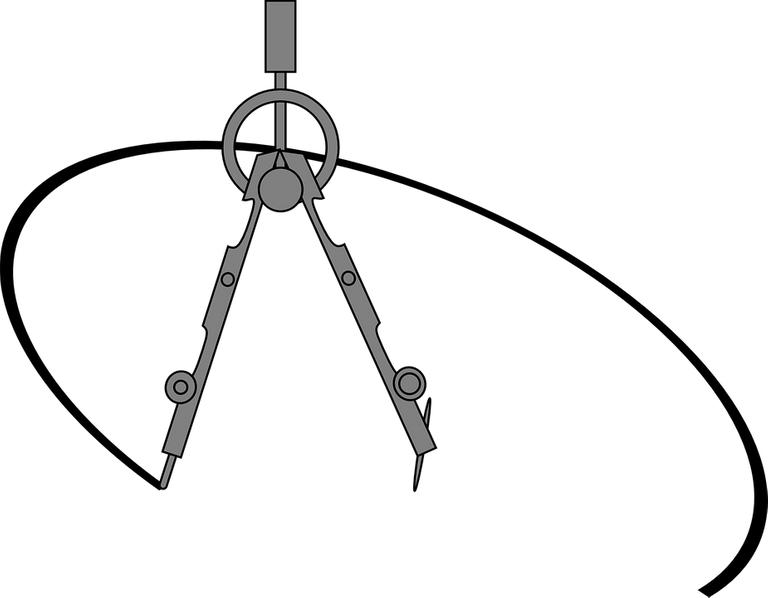[Image1]

## Introduction

Hey it's a me again @drifter1!

This is the fifth part of my high-school refresher series on Geometry. I highly suggest checking out part1, part2, part3 and part4 before this part.

So, without further ado, let's get straight into it!

## Solid Geometry and 3-Dimensional Space (3D)

Solid Geometry is all about 3 Dimensions. 3D space is defined by the following three dimensions:

• Width
• Height
• Depth

### 3D Cartesian Coordinates

When using Cartesian Coordinates, those three dimensions yield the the x, y and z axes respectively, but not necessarily in that exact order! For example, a common 3D Cartesian Coordinate System is shown below.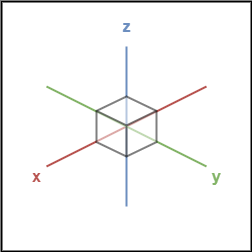### Common 3D Shapes

Some common 3D Shapes (or Solids) are shown below.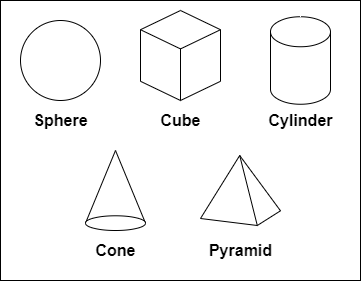## Solid Properties

Solids have specific properties, which are basically things that are unique and special to each and everyone of them. Those are:

• Volume : How much 3D space the solid occupies (Think of how much water that can be stored within)
• Surface Area : How much the total outside area of the solid occupies (Think of how much paint would be needed to cover the outside)
• Vertices : The corner points of the solid
• Faces : The single flat surfaces of the solid
• Edges : The line segments between the faces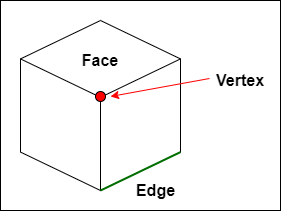Let's cover some of the common 3D shapes.

### Cube

A Cube has:

• 6 Faces
• 8 Vertices
• 12 Edges

The volume is calculated as x3, where x is the length of an edge. The surface area is 6 times the area of a square, as each face is basically a 2D square, and thus given by 6x2.

### Cuboid

A Cuboid has the same amount of faces, vertices and edges as a cube, but the lengths are now x, y and z, and not equal. The volume of a cuboid is simply xyz, the multiplication of the three edges. The surface area is 2xy + 2xz + 2yz, and so again the sum of the individual areas of the 6 rectangular shapes, which come in opposite-to-each-other pairs.

### Sphere

A Sphere has no faces, vertices or edges (its not a Polyhedron as we will see later on). The volume and surface area are given by: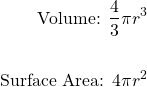where r is the radius of the sphere.

### Cylinder

A Cylinder has:

• No Vertices
• 2 Edges
• 3 Faces

The edges are curved and wrapping around the circular faces on the top and bottom of the shape. The faces are the two circles on the top and bottom, as well as the curved surface which wraps around them.

The volume and surface are given by: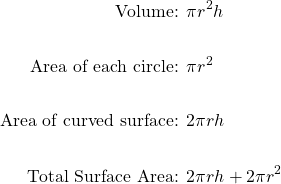where r is the radius of the circular faces and h is the height of the cylinder.

### Pyramid

A Pyramid is made by connecting a base to an apex point. The base can be any polygon, and the remaining faces need to be triangles. If the base is a triangle we talk about a triangular pyramid, if it's a square about a square pyramid (like the pyramids in Egypt) etc.

If the apex point is directly above the center of the base the pyramid is considered a right pyramid, otherwise its an Oblique pyramid.

When the base is a regular polygon, the pyramid is also considered regular, otherwise it's irregular.

The volume of a pyramid is 1/3 of the base area times the height. If all side faces are equal, the surface area is the base area + half the perimeter times the slant length. The slant length is the distance from the center of an edge towards the apex points. If the sides are different (irregular triangle) then the area is generalized into base area + lateral area, where the lateral area will be equal to the sum of the areas of each individual side triangle.

## Polyhedra and Non-Polyhedra

Any solid that has only flat faces is considered a Polyhedron. In other words, each face must be a polygon. Solids with at least one curved surface are known as Non-Polyhedra. For example, a cube is considered a Polyhedron as all faces are squares, but a cylinder is not, as it contains a non-polygon face.

### Euler's Formula

When counting the faces, vertices and edges of a solid, one interesting equation to consider is Euler's formula, which applies only to Polyhedra and convex shapes.

If F is the number of faces, V the number of vertices and E the number of edges then:

F + V - E = 2

For example, in the case of a cube:

6 + 8 - 12 = 2

### Platonic Solids

Special types of solids, which are also Polyhedra, are the five platonic solids. For them each face is a regular polygon, and the same number of polygons meets at each vertex. More specifically, the platonic solids are:

• Tetrahedron :
• 3 Triangles meet at each vertex
• 4 Faces, 4 Vertices and 6 Edges
• Cube :
• 3 Squares meet at each vertex
• 6 Faces, 8 Vertices and 12 Edges
• Octahedron :
• 4 Triangles meet at each vertex
• 8 Faces (Octo / Οκτώ in Greek means 8), 6 Vertices and 12 Edges
• Dodecahedron :
• 3 Pentagons (five sides) meet at each vertex
• 12 Faces (Dodeca / Δώδεκα in Greek means 12), 20 Vertices and 30 Edges
• Icosahedron :
• 5 Triangles meet eat each vertex
• 20 Faces (Icosi / Είκοσι in Greek means 20), 12 Vertices and 30 Edges[Image 2]

### Prisms

Another interesting type of solid is the Prism, with two identical ends, only flat faces and the same cross-section along its length. For example, a Triangular Prism has two identical triangles in the two ends, and the cross-section is also a triangle. Similarly, we can define the Square Prism, Pentagonal Prism etc, and interestingly enough a Cube is also a prism, because it's a square all along its length.

When the sides and cross-section are regular polygons, the prism is also called regular, otherwise it's irregular. When the two ends are perfectly aligned the prism is called right, otherwise oblique.

The Surface area of a prism is 2 times the base area + base perimeter times the length.

The Volume is equal to the base area times the length.

The surface area and volume are still the same for oblique prisms, as "leaning over" doesn't affect the height, as the height (or length) is calculated in a right angle from the base.

## RESOURCES:

### Images

Mathematical equations used in this article, have been generated using quicklatex.

Block diagrams and other visualizations were made using draw.io.

## Final words | Next up

And this is actually it for today's post!

Next time we will dive into some more advanced topics...and that part might also be the last one!

Also, currently, other ideas for "All About" articles that I have in mind include:

• Polynomial Arithmetic
• Exponentials and Logarithms
• Rational Expressions

Basically more High-School Math Refreshers!

See ya!Keep on drifting!